## Quiz 3 : Demand, Supply, and Market EquilibriumLooking for Economics Homework Help?# Quiz 3 : Demand, Supply, and Market Equilibrium

(a) We are given the following table with missing information to fill out.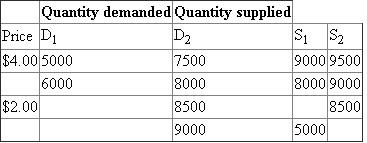We are given that if demand is D1 and supply is S1 , then the equilibrium quantity is 7000 gallons per month. Looking at the information for D1 and S1 , the only price at which this can happen is \$2.00 because we already have quantity supplied for all other prices, and at the equilibrium, the quantity supplied must equal the quantity demanded at some price. So, we can fill in 7000 gallons per month for the price of \$2.00.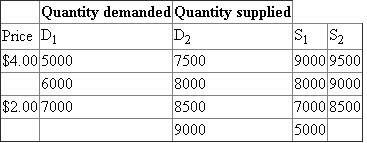When demand is D2 and supply is S1 , we are given that the equilibrium price is \$3.00. We know that at the equilibrium price, the quantity demanded is equal to the quantity supplied. Here, the equilibrium when the demand is D2 and supply is S1 happens at a quantity of 8000 because both quantities supplied and quantity demanded is 8000. This means that the corresponding equilibrium price is \$3.00.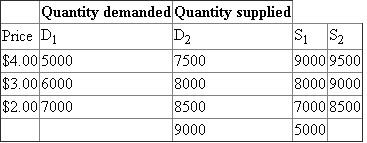When demand is D2 and supply is S1 , we are given that there is an excess demand of 4000 gallons per month at a price of \$1.00 per gallon. Here, the last row for price must be \$1.00 because the quantity demanded is 9000 and the quantity supplied is 5000, which gives us the excess demand of 4000 gallons per month.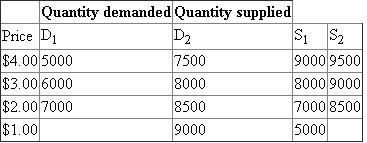Finally, we are given that if demand is D1 and supply is S2 , the equilibrium quantity is 8000 gallons per month. Since quantity demanded must equal quantity supplied in the equilibrium, so, when demand is D1 and supply is S2 , then the equilibrium must be at \$1.00 because at all other prices, the quantity demanded is not equal to the quantity supplied. Therefore, we fill in the last two cells with 8000 gallons per month.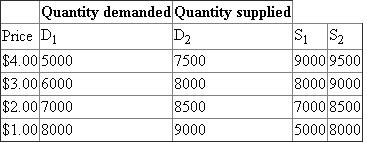(b) At the equilibrium when the demand is D1 and supply is S1 , the equilibrium quantity is 7000 gallons per month at a price of \$2.00. When the demand is D1 and the supply is S2 , the equilibrium quantity is 8000 gallons per month at a price of \$1.00. Hence, the equilibrium quantity goes up by 1000 gallons per month and the equilibrium price goes down by \$1.00 when the supply curve shifts from S1 to S2. (c) If we start from the equilibrium when the supply is S2 and demand is D2 , we find that the equilibrium quantity is 8500 gallons per month at a price of \$2.00. Now, if both curve shifted, the new equilibrium quantity (where quantity demanded is equal quantity supplied) will be 7000 gallons per month, at a price of \$2.00. We see here that the equilibrium price stayed the same. If only supply falls, then the equilibrium quantity is 8000 gallons per month at a price of \$3.00. The equilibrium price went up when only supply falls. If only demand falls i.e. when demand is D1 and supply is S2 , then the equilibrium quantity is 8000 gallons per month at the equilibrium price of \$1.00. Thus, if only demand falls, the equilibrium price falls. (d) When supply is S1 and demand is D1 , for the price of \$3.00 to be an equilibrium price then, the quantity demanded and quantity supplied must be equal. However, quantity demanded is 6000 gallons per month and quantity supplied is 8000 gallons per month. Therefore, to make \$3.00 the equilibrium price, demand must increase by 2000 gallons per month so that the quantity demanded will be equal to the quantity supplied at 8000 gallons per month. When supply is S1 and demand is D1 , for the price of \$4.00 to be an equilibrium price, then, the quantity demanded and quantity supplied must be equal. However, quantity demanded is 5000 gallons per month and quantity supplied is 9000 gallons per month. Therefore, to make \$4.00 the equilibrium price, demand must increase by 4000 gallons per month so that the quantity demanded will be equal to the quantity supplied at 9000 gallons per month.

Shortages or surpluses are more likely with preset prices, such as those on tickets, than flexible prices such as those on gasoline because the preset prices are set on the basis of some estimate about future demand or the present capacity so the actual demand and supply situations may differ from their expected values, creating either a surplus or shortage in the market. On the other hand, flexible prices are determined on the basis of the developments on the demand side and the supply side, in addition to their relative strength. In other words, prices are free to be determined on the basis of the real developments, so the equilibrium prices and quantities are always maintained.

(a) We are given a table with some values missing (missing values denoted A, B, C, D, and E)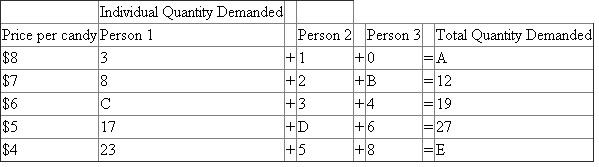First, note that the total quantity demanded is simply the sum of all individual demand in the economy. In this sample economy with only three people, the sum of each individual demand then is equal to the total quantity demanded. So, Person 1's demand + Person 2's demand + Person 3's demand = total quantity demanded of the economy. To find A and E, we simply have to add up the quantity demanded of each individual (person 1, person 2, and person 3) to get the total quantity demanded. That means A=3+1+0, which means A=4, and E=23+5+8, which means E=36. We can also find B, C, and D by using the fact that the sum of the three individual quantity demanded must equal the total quantity demanded. To find B, since at each price, Person 1's demand + Person 2's demand + Person 3's demand = total quantity demanded, this also means that total quantity demanded - Person 1's demand - Person 2's demand = Person 3's demand. So, 8+2+B=12, which means B=12-8-2 or B=2. Similarly, C+3+4=19, so C=19-4-3 or C=12. Finally, 17+D+6=27, so D=27-17-6 or D= 4. Putting in the values that we just calculated, we now have: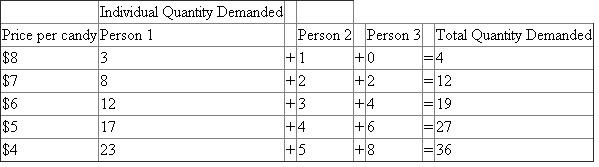(b) At a price of \$5, Person 1 demandS17 units, Person 2 demands 4 units, and Person 3 demands 6 units. This means that the person who demands the least is Person 2. At a price of \$7, Person 1 demands 8 units, Person 2 demandS2 units, and Person 3 demandS2 units. This means that the person who demands the most is Person 1. (c)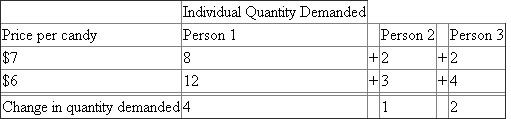When the price is lowered from \$7 to \$6, we can calculate the change in quantity demanded of each person, as shown above. We see that the person who had the biggest change in quantity demanded is Person 1, which a change in quantity demanded of 4. (d) One of the shifters of the demand curve is the number of buyers. The idea is that if we took out a person, say Person 1, the total quantity demanded will be lower at each price level. That means that the demand curve will shift to the left (the demand curve shifting to the left means that at every single price level, the total quantity demanded is lower than it was before the shift). If Person 2 doubled his purchases at each possible price, this will cause the total quantity demanded to be higher at each price level. This is exactly what a rightward shift of the demand curve signifies, that the quantity demanded at each price level is higher than it was before the shift. (e) If the total quantity demanded at \$6 increases from 19 to 38, then this is a change in demand. A change in the quantity demanded would be when we go from one price to another and see that the total quantity demanded changes. For example, going from \$8 to \$7 meant going from a total quantity demanded of 4 to 12. However, a change in demand happens when at the same price, there is a higher (or lower) total quantity demanded, which is what we have here when at \$6, the total quantity demanded went from 19 to 38.

There is no answer for this question

There is no answer for this question

There is no answer for this question